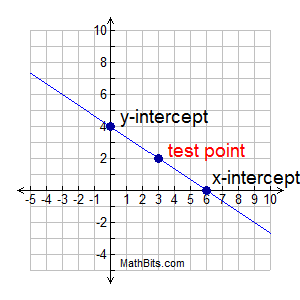Graphing Linear Equations MathBitsNotebook.com Terms of Use   Contact Person: Donna RobertsYou have already seen how to graph a linear equation by using a table (chart),
or by using the slope-intercept method .
Now, let's take a look at another graphing method.An Intercept Graphing Method:

Graph 2x + 3y = 12
Yes, you can re-write this equation and use a table or the slope-intercept method for graphing. But, there is another option which may be easier to use in this situation.

We are going to obtain the x-intercept and the
y-intercept. We will then choose one additional test point (to avoid possible errors) and show that all three points form a line.

 Remember: to find the y-intercept, set x = 0. to find the x-intercept, set y = 0.If you want to organize your work,
you can set up a small table or chart.

 x y 0 0 ?

The first slot is for the
y
- intercept
(where x = 0).
The second slot is for the
x
- intercept
(where y = 0).
The third slot is for any test point you choose to plot to check for errors. (Hint: choose an x-value that will fit easily into the equation.)

 This graphing approach is particularly useful when your equation is in standard form, Ax + By = C.

Here is the completed chart
for the graph seen above.

 x y 0 4 6 0 3 2
y-intercept - set x = 0
2•0 + 3y = 12
3y = 12 and y = 4

x-intercept - set y = 0
2x + 3•0 = 12
2x = 12 and x = 6

We chose x = 3 to create the test point.
 2•3 + 3y = 12 6 + 3y = 12 3y = 6 y = 2 The fact that these three points form a straight line on the graph tells us that we did not make an error.Using the graphing calculator:

• We have seen the graphing calculator at work in graphing lines of the form y = mx + b.

• Since most graphing calculators require that equations be entered in "y=" form, they have a problem graphing vertical lines, such as x = 4. The third box listed below, will show you a trick to get your graphing calculator to deal with a vertical line without having to use the DRAW command.

• The graphing calculator can also create the equation of a line given two points on the line. The calculator uses a statistics feature called a "Linear Regression" to give you the slope and y-intercept of y = ax + b (the calculator's version of y = mx + b). Follow the link in the last box below to see how this works.For calculator help with Graphing click here.For calculator help with Graphing Tidbits click here.Calculator help with Graphing Vertical Liness click here.For calculator help with Creating Equations click here.